# please answer following question 3. For the cell diagram below write out and balance the reaction...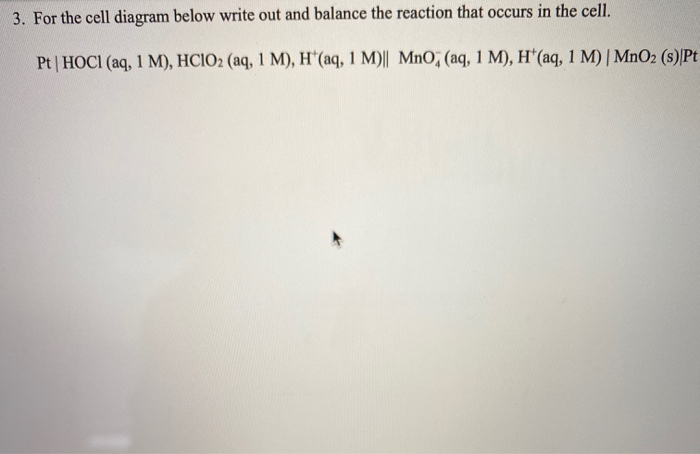please answer following question
3. For the cell diagram below write out and balance the reaction that occurs in the cell. Pt | HOCI (aq, 1 M), HCIO2 (aq, 1 M), H*(aq, 1 M)|| Mno; (aq, 1 M), H(aq, 1 M) | MnO2 (s)Pt

While reading a cell notation its important to remember the Formula 'ABC' (Anode.....Bridge.......Cathode)The first hald is the reaction at anodic half, its an oxidation half constinting of an oxidation reaction while the other reaction is in the cathodic half and its the reduction reaction

Anode: (oxidation half)

2HClO2 + 4H+ + 4e = 2HOCl + 2H2O

Cathode: (reduction half)

4 H+ + 3 MnO4- = 2 H2O + MnO2 + 4e-

#### Earn Coin

Coins can be redeemed for fabulous gifts.

Similar Homework Help Questions
• ### 14. Balance each reaction below, and write a cell schematic representing the reaction as it would occur in a galvan...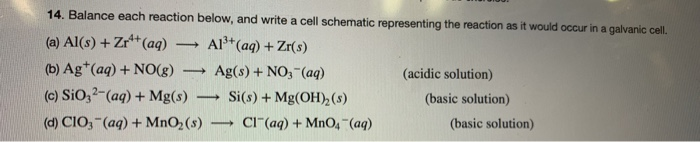14. Balance each reaction below, and write a cell schematic representing the reaction as it would occur in a galvanic cell. (a) Al(s) +Zrº+ (aq) - Al+(aq) + Zr(s) (6) Ag+ (aq) + NO(g) Ag(s) + NO3- (aq) (acidic solution) (c) Si0,2- (aq) + Mg(s) — Si(s) + Mg(OH),(s) (basic solution) (d) CIO3- (aq) + MnO2 (s) — Cl(aq) + MnO4- (aq) (basic solution)

• ### selected answer is incorrect Question 2 0 / 1 point Balance the following reaction which occurs...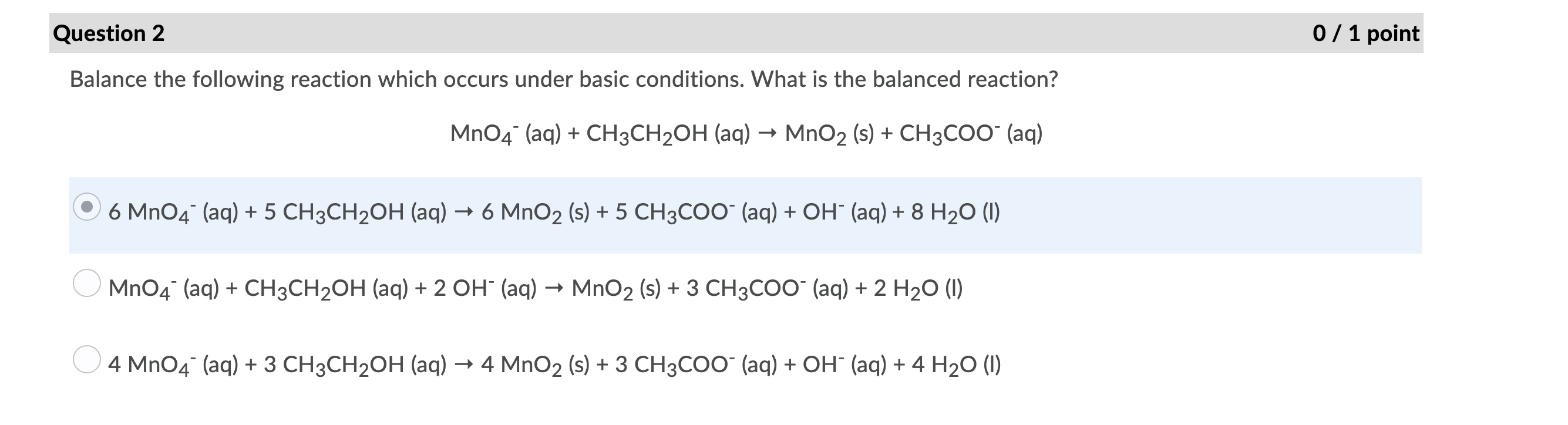selected answer is incorrect Question 2 0 / 1 point Balance the following reaction which occurs under basic conditions. What is the balanced reaction? MnO4 (aq) + CH3CH2OH (aq) → MnO2 (s) + CH3COO- (aq) 6 MnO4- (aq) + 5 CH3CH2OH (aq) + 6 MnO2 (s) + 5 CH3COO- (aq) + OH- (aq) + 8 H20 (1) MnO4 (aq) + CH3CH2OH (aq) + 2 OH- (aq) + MnO2 (s) + 3 CH3COO- (aq) + 2 H20 (1) 4 MnO4 (aq)...

• ### Choose the correct QUESTION 19 According to the following cell notation, the species that is undergoing...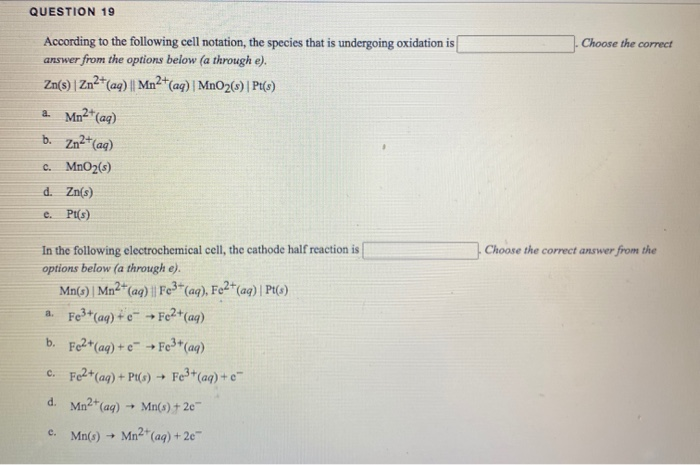Choose the correct QUESTION 19 According to the following cell notation, the species that is undergoing oxidation is answer from the options below (a through e). Zn(s) Zn2+ (aq) || Mn2+(aq)|MnO2(3)| Pt() a. Mn2+ (aq) b. Zn2+ (aq) c. MnO2(3) d. Zn(s) e. Pt(s) Choose the correct answer from the In the following electrochemical cell, the cathode half reaction is options below (a through e). Mn(s) Mn2+ (aq) || Fe3+ (aq), F ), Fe2+ (aq)| Pt() 4. Fe3+(aq) + 6...

• ### please answer all parts to this question! They are all from one question, so they could...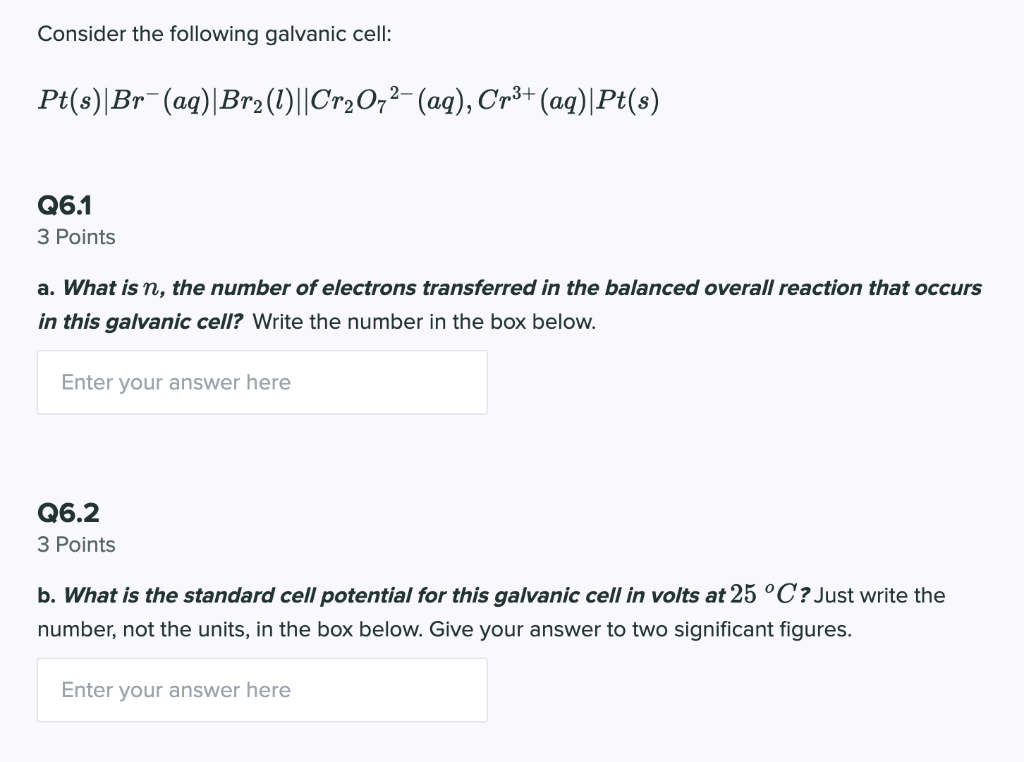please answer all parts to this question! They are all from one question, so they could not be posted separately. Please double check your answer! The last person got it wrong :( thanks! Will give thumbs up. Consider the following galvanic cell: Pt(s)|Br- (aq)|Br2(1)||Cr2O72- (aq), Cr3+ (aq)|Pt(s) Q6.1 3 Points a. What is n, the number of electrons transferred in the balanced overall reaction that occurs in this galvanic cell? Write the number in the box below. Enter your answer...

• ### For each of the following galvanic cells (1) write out the conventional (overall) cell reaction and...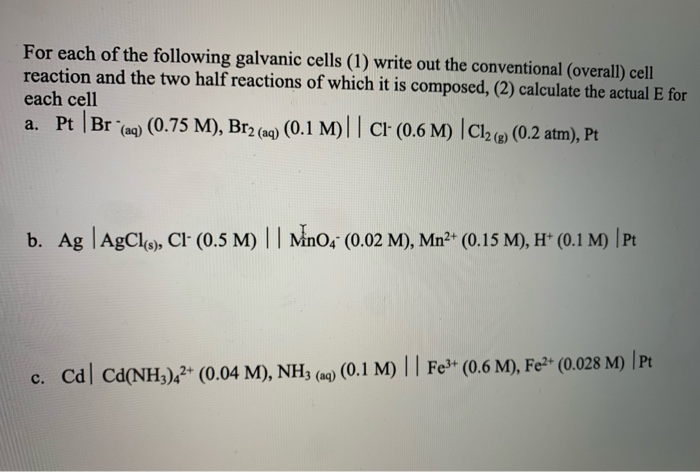For each of the following galvanic cells (1) write out the conventional (overall) cell reaction and the two half reactions of which it is composed, (2) calculate the actual E for each cell a. Pt Br (aq) (0.75 M), Br2 (aq) (0.1 M)||Ci' (0.6 M) Cl2(Q) (0.2 atm), Pt b. Ag AgCl(), Cl- (0.5 M) || MinO4 (0.02 M), Mn2+ (0.15 M), H* (0.1 M) | Pt c. Cd Ca(NH3)42+ (0.04 M), NH3 (aq) (0.1 M) || Fe3+ (0.6 M),...

• ### Question 4 (1 point) Write the cell diagram for the reaction 2AgCl(s) + H2(g) --> 2Ag(s)...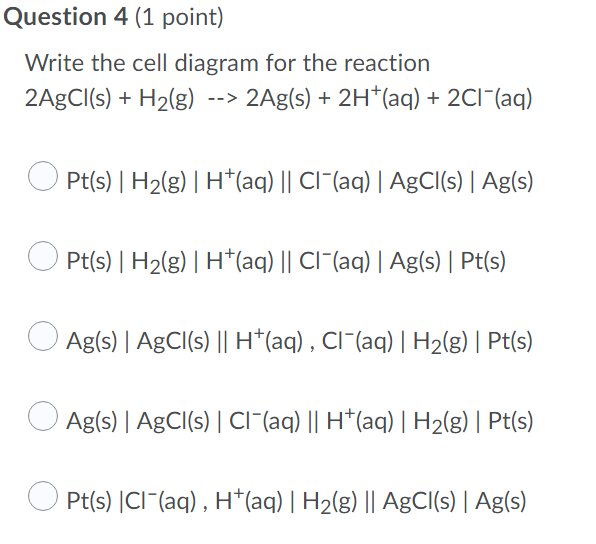Question 4 (1 point) Write the cell diagram for the reaction 2AgCl(s) + H2(g) --> 2Ag(s) + 2H+(aq) + 2C1-(aq) OPt(s) | H2(g)| H+(aq) || CI+(aq)| AgCl(s) | Ag(s) Pt(s) | H2(g)| H+(aq) || CI+(aq) | Ag(s) | Pt(s) O Ag(s) | AgCl(s) || H+(aq), Ci-(aq) | H2(g) | Pt(s) O Ag(s) | AgCl(s) | CI+(aq) || H+(aq)| H2(g) | Pt(s) Pt(s) Cl(aq), H(aq)| H2(g) || AgCl(s) Ag(s)

• ### please answer all parts to this question! they are all part of one question so i...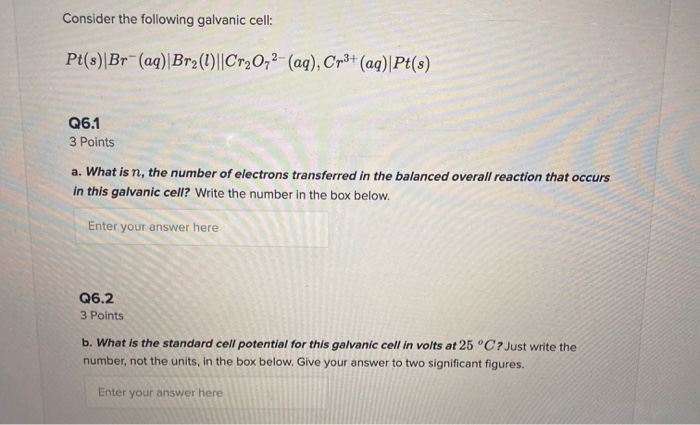please answer all parts to this question! they are all part of one question so i could not post them separately. will give thumbs up! should be parts a through d! thanks. Consider the following galvanic cell: Pt(s)|Br-(aq) Br2(1)||Cr20,2- (aq), Cr3+ (aq)|Pt(s) Q6.1 3 Points a. What is n, the number of electrons transferred in the balanced overall reaction that occurs in this galvanic cell? Write the number in the box below. Enter your answer here Q6.2 3 Points b....

• ### A galvanic cell is powered by the following redox reaction: 3 Fe'*(aq) + MnO(s) + 4...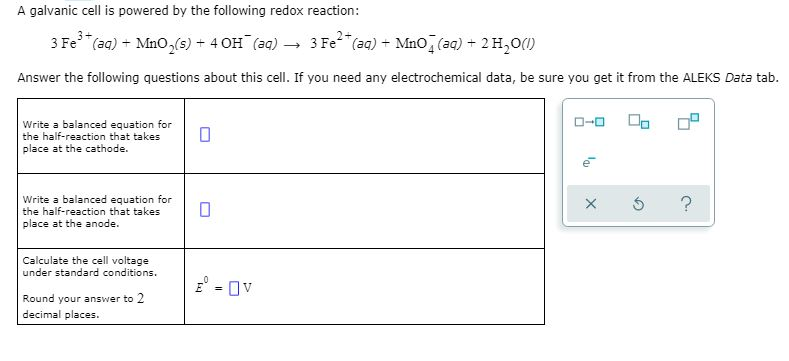A galvanic cell is powered by the following redox reaction: 3 Fe'*(aq) + MnO(s) + 4 OH(aq) → 3 Fe2+ (aq) + Mno (aq) + 2 H,00 Answer the following questions about this cell. If you need any electrochemical data, be sure you get it from the ALEKS Data tab. Write a balanced equation for the half-reaction that takes place at the cathode. Write a balanced equation for the half-reaction that takes place at the anode. x 5 ? Calculate...

• ### please answer all questions for rate. thank you :) For the following equation: MnO4 (aq) +...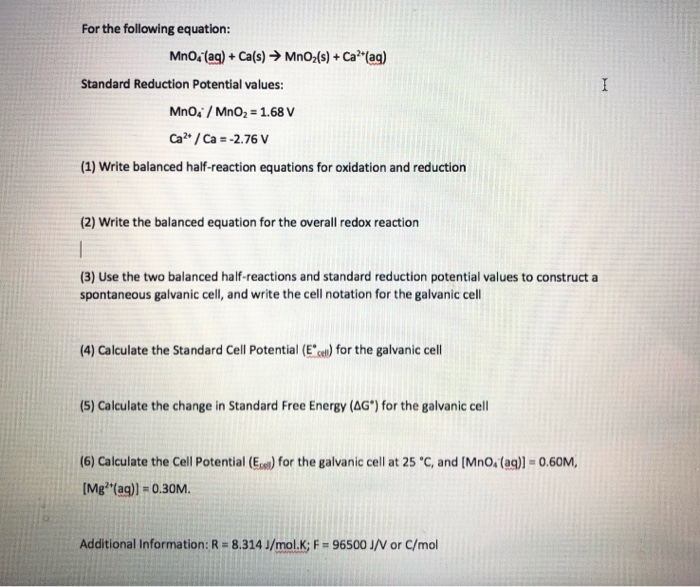please answer all questions for rate. thank you :) For the following equation: MnO4 (aq) + Ca(s) → MnO2(s) + Ca?*(aq) Standard Reduction Potential values: Mno. MnO2 = 1.68 V Ca? / Ca = -2.76 V (1) Write balanced half-reaction equations for oxidation and reduction (2) Write the balanced equation for the overall redox reaction (3) Use the two balanced half-reactions and standard reduction potential values to construct a spontaneous galvanic cell, and write the cell notation for the galvanic...

• ### please consider the reaction below Fe^2+(aq)+MnO-4(aq)>Fe^3+(aq)+Mn^2+(aq) write the oxidation half-reaction and balance write the reduction half-reaction...

please consider the reaction below Fe^2+(aq)+MnO-4(aq)>Fe^3+(aq)+Mn^2+(aq) write the oxidation half-reaction and balance write the reduction half-reaction and balance combine both half-reactions and balance